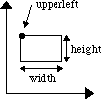## 3.1 Coordinates

Figure 4: Coordinate SystemWhen a graphical object is to be drawn, the points that defines the outline of the graphical object must be specified in some way, i.e. where is the shape supposed to be. Bifrost uses standard Cartesian coordinates for this purpose. Standard Cartesian coordinate subtraction and addition are supported. The Figure above illustrates the coordinate system used.

As shown, an axis-parallel rectangle is described by one point (upperleft) and two integers (width and height).

 The Bifrost Graphics System - Reference Manual © 1991-2004 Mjølner Informatics [Modified: Tuesday October 24th 2000 at 15:02]